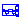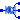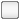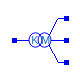## OpenIPSL.Electrical.ThreePhase.Branches.MonoTri

Models for hybrid positive-sequence/three-phase devices

### Information

This package contains models for hybrid positive-sequence/three-phase devices, and a set of functions used to calculate three-phase impedance matrices.

Extends from Modelica.Icons.Package (Icon for standard packages).

### Package Content

Name DescriptionLine_MT Transmission Line modeled as a PI element with a hybrid interface positive-sequence/three-phaseTransformer_MT Transformer modeled in a PI element with a hybrid interface positive-sequence/three-phaseMonoTriFcn Set of functions used in hybrid devices when finite Norton equivalent impedances are usedTransformerFcn Set of functions used to calculate hybrid positive-sequence/three-phase impedance matrices for transformersLineFcn Set of functions used to calculate hybrid positive-sequence/three-phase impedance matrices for lines

##OpenIPSL.Electrical.ThreePhase.Branches.MonoTri.Line_MT

Transmission Line modeled as a PI element with a hybrid interface positive-sequence/three-phase

### Information

This model was design to represent a hybrid positive-sequence/three-phase power line.

The user should input the series conductance and susceptance, and half shunt susceptance (line charging). All in matrix form, since this model can be used to represent unbalanced lines. Series conductance (Gser) matrix is considered to have the following structure:

[Gseraa, Gserab, Gserac;

Gserab, Gserbb, Gserbc;

Gserac, Gserbc, Gsercc]

Series susceptance (Bser) matrix is considered to have the following structure:

[Bseraa, Bserab, Bserac;

Bserab, Bserbb, Bserbc;

Bserac, Bserbc, Bsercc]

The series admittance matrix is Yser = Gser+jBser. Each of the two shunt susceptance (Bsht) matrices is considered to have the following structure:

[Bshtaa, Bshtab, Bshtac;

Bshtab, Bshtbb, Bshtbc;

Bshtac, Bshtbc, Bshtcc]

In addition to that, the user should also state if the hybrid interface should be calculated in an approximate or exact way. If the exact way is selected, the user should also input Norton equivalent admittances for positive, negative and zero sequences calculated in the point of interconnection of the hybrid device. If approximate model is selected, these admittances are set to be zero. Based on the set of parameters selected by the user, the pi-equivalent impedance matrices are calculated and the line equation is assembled.

The positive-sequence system is connected using pin p, while three-phase system is connected using pins A, B, and C

Extends from Branches.BaseClasses.baseLine (Partial base power line model).

### Parameters

NameDescription
Power flow data
SNominal Power [V.A]
fSystem Frequency [Hz]
Selection of model
ModelType0- Assuming that the negative and zero norton admittances are infinite (Approximation), 1- Considering that the negative and zero norton admittances finite values;
Parameters for an 'abc'-Model
GseraaElement (1,1) in series conductance matrix 
BseraaElement (1,1) in series susceptance matrix 
GserabElement (1,2) in series conductance matrix 
BserabElement (1,2) in series susceptance matrix 
GseracElement (1,3) in series conductance matrix 
BseracElement (1,3) in series susceptance matrix 
GserbbElement (2,2) in series conductance matrix 
BserbbElement (2,2) in series susceptance matrix 
GserbcElement (2,3) in series conductance matrix 
BserbcElement (2,3) in series susceptance matrix 
GserccElement (3,3) in series conductance matrix 
BserccElement (3,3) in series susceptance matrix 
BshtaaElement (1,1) in shunt half susceptance matrix 
BshtabElement (1,2) in shunt half susceptance matrix 
BshtacElement (1,3) in shunt half susceptance matrix 
BshtbbElement (2,2) in shunt half susceptance matrix 
BshtbcElement (2,3) in shunt half susceptance matrix 
BshtccElement (3,3) in shunt half susceptance matrix 
Norton equivalent admittances in terminal K - Considering the negative and zero norton admittances have finite values
G_0Zero-sequence Norton equivalent conductance 
B_0Zero-sequence Norton equivalent susceptance 
G_1Positive-sequence Norton equivalent conductance 
B_1Positive-sequence Norton equivalent susceptance 
G_2Negative-sequence Norton equivalent conductance 
B_2Negative-sequence Norton equivalent susceptance 

NameDescription
p
A
B
C

##OpenIPSL.Electrical.ThreePhase.Branches.MonoTri.Transformer_MT

Transformer modeled in a PI element with a hybrid interface positive-sequence/three-phase

### Information

This model was design to represent a hybrid positive-sequence/three-phase two-winding transformer.

The user should input the copper resistance R and leakage reactance X in per unit values. The user should also input the tap value, which is the relation between primary and secondary voltage levels, but in per unit. The user should select the transformer three-phase connection and, in addition to that, the user should also state if the hybrid interface should be calculated in an approximate or exact way. If the exact way is selected, the user should also input Norton equivalent admittances for positive, negative and zero sequences calculated at the point of interconnection of the hybrid device. If approximate model is selected, these admittances are set to be zero. Based on the set of parameters selected by the user, the pi-equivalent impedance matrices are calculated and the transformer equation is assembled. The transformer does not take into account excitation branch, since it is often neglected in transient-stability studies.

Primary side, modeled as positive-sequence equivalent, is represented by pin p. Secondary side, modeled as full three-phase system, is represented by pins A, B, and C

### Parameters

NameDescription
Connection0 Yg-Yg; 1 D-D; 2 Y-Y; 3 D-Yg; 4 Yg-D; 5 D-Y; 6 Y-D; 7 Y-Yg; 8 Yg-Y;
Selection of model
ModelType0- Assuming that the negative and zero norton admittances are infinite (Approximation), 1- Considering that the negative and zero norton admittances finite values;
Transformer parameters
tapNominal tap ratio (Vs/Vp)
XLeakage reactance 
RWindings copper resistance 
Norton equivalent admittances in terminal K - Considering that the negative and zero norton admittances have finite values
G_0Zero-sequence Norton equivalent conductance 
B_0Zero-sequence Norton equivalent susceptance 
G_1Positive-sequence Norton equivalent conductance 
B_1Positive-sequence Norton equivalent susceptance 
G_2Negative-sequence Norton equivalent conductance 
B_2Negative-sequence Norton equivalent susceptance 

### Connectors

NameDescription
p
A
B
C
Automatically generated Mon Oct 10 14:35:56 2022.